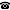# 2014 seminar talk: Monotone metric spaces

Talk held by Jonathan Verner (Charles University, Prague, Czech Republic) at the KGRC seminar on 2014-11-13.

### Abstract

A metric space is ($k$-)monotone, if there is a linear order on it such that for any three points $x < y < z$ the distance between $x$ and $y$ is at most $k$ times the distance between $x$ and $z$. A space is $\sigma$-monotone if it is the union of countably many monotone spaces. Hrušák and Zindulka asked, whether there is (in ZFC) a non $\sigma$-monotone space of size $\aleph_1$. We investigate this and related questions.

Kurt Gödel Research Center for Mathematical Logic. Währinger Straße 25, 1090 Wien, Austria.+43-1-4277-50501. Last updated: 2010-12-16, 04:37.